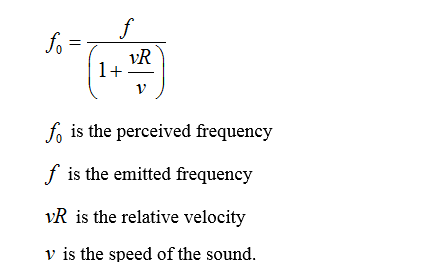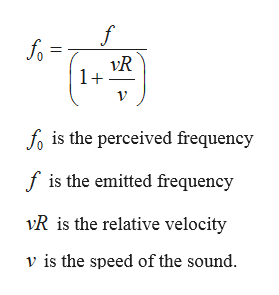# The horn of a train has a frequency of 375Hz. If the train is moving away from you at 38m/x, then what frequency (in Hz) do you hear? Assume the speed of sound is 343m/s.

Question
1 views

The horn of a train has a frequency of 375Hz. If the train is moving away from you at 38m/x, then what frequency (in Hz) do you hear? Assume the speed of sound is 343m/s.

check_circle

Step 1

Using Doppler effect, when the source is moving away from the person,...help_outlineImage Transcriptionclose1+ fo is the perceived frequency f is the emitted frequency vR is the relative velocity v is the speed of the sound. fullscreen

### Want to see the full answer?

See Solution

#### Want to see this answer and more?

Solutions are written by subject experts who are available 24/7. Questions are typically answered within 1 hour.*

See Solution
*Response times may vary by subject and question.
Tagged in

### Physics